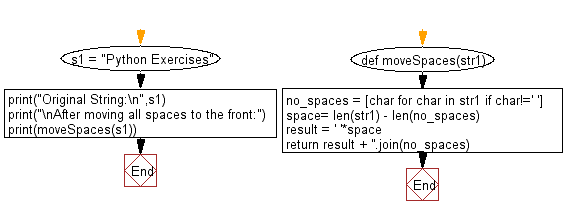﻿ Python: Move all spaces to the front of a given string in single traversal - w3resource# Python: Move all spaces to the front of a given string in single traversal

## Python String: Exercise-71 with Solution

Write a Python program to move all spaces to the front of a given string in single traversal.

Sample Solution:-

Python Code:

``````def moveSpaces(str1):
no_spaces = [char for char in str1 if char!=' ']
space= len(str1) - len(no_spaces)
# Create string with spaces
result = ' '*space
return result + ''.join(no_spaces)

s1 = "Python Exercises"
print("Original String:\n",s1)

print("\nAfter moving all spaces to the front:")
print(moveSpaces(s1))
```
```

Sample Output:

```Original String:
Python Exercises

After moving all spaces to the front:
PythonExercises
```

Pictorial Presentation:Flowchart:## Visualize Python code execution:

The following tool visualize what the computer is doing step-by-step as it executes the said program:

Python Code Editor:

Have another way to solve this solution? Contribute your code (and comments) through Disqus.

What is the difficulty level of this exercise?

Test your Programming skills with w3resource's quiz.

﻿

## Python: Tips of the Day

What is the difference between Python's list methods append and extend?

append: Appends object at the end.

```x = [1, 2, 3]
x.append([4, 5])
print (x)
```

Output:

```[1, 2, 3, [4, 5]]
```

extend: Extends list by appending elements from the iterable.

```x = [1, 2, 3]
x.extend([4, 5])
print (x)
```

Output:

```[1, 2, 3, 4, 5]
```

Ref: https://bit.ly/2AZ6ZFq